# Verbal Reasoning - Dice

### Exercise :: Dice - Dice 1

11.

Here 4 positions of a cube are shown. Which sign will be opposite to '+' ?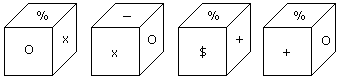A. % B. - C. x D. \$

Explanation:

From position I and III common face with % is in the same position. Hence according to rule (3) opposite is X.

12.

Two positions of a cubical block are shown. When 5 is at the top which number will be at bottom?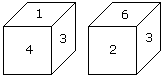A. 1 B. 2 C. 3 D. 4

Explanation:

In these 2 positions one common face with number 3, is in same position. Hence according to rule (3), 1 is opposite to 6 and 4 is opposite to 2. Therefore 5 is opposite to 3.

13.

From the four positions of a dice given below, find the color which is opposite to yellow ?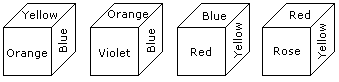A. Violet B. Red C. Rose D. Blue

Explanation:

The colours adjacent to yellow are orange, blue, red and rose. Hence violet will be opposite to yellow.

14.

When the digit 5 is on the bottom then which number will be on its upper surface?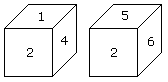A. 1 B. 3 C. 4 D. 6

Explanation:

According to the rule no. (3), common faces with number 2 are in same positions. Hence when the digit 5 is on the bottom then 1 will on the upper surface.

15.

How many points will be on the face opposite to the face which contains 3 points?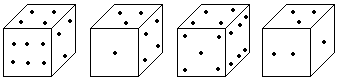A. 2 B. 4 C. 5 D. 6